# HSSlive: Plus One & Plus Two Notes & Solutions for Kerala State Board

## AP Board Class 7 Maths Chapter 7 Data Handling Ex 1 Textbook Solutions PDF: Download Andhra Pradesh Board STD 7th Maths Chapter 7 Data Handling Ex 1 Book AnswersAP Board Class 7 Maths Chapter 7 Data Handling Ex 1 Textbook Solutions PDF: Download Andhra Pradesh Board STD 7th Maths Chapter 7 Data Handling Ex 1 Book Answers

## Andhra Pradesh State Board Class 7th Maths Chapter 7 Data Handling Ex 1 Books Solutions

 Board AP Board Materials Textbook Solutions/Guide Format DOC/PDF Class 7th Subject Maths Chapters Maths Chapter 7 Data Handling Ex 1 Provider Hsslive

2. Click on the Andhra Pradesh Board Class 7th Maths Chapter 7 Data Handling Ex 1 Answers.
3. Look for your Andhra Pradesh Board STD 7th Maths Chapter 7 Data Handling Ex 1 Textbooks PDF.
4. Now download or read the Andhra Pradesh Board Class 7th Maths Chapter 7 Data Handling Ex 1 Textbook Solutions for PDF Free.

## AP Board Class 7th Maths Chapter 7 Data Handling Ex 1 Textbooks Solutions with Answer PDF Download

Find below the list of all AP Board Class 7th Maths Chapter 7 Data Handling Ex 1 Textbook Solutions for PDF’s for you to download and prepare for the upcoming exams:

Question 1.
Maximum day time temperatures of Hyderabad in a week (from 26th February to 4th March, 2011) are recorded as 26°C, 27°C, 30°C, 30°C, 32°C, 33°C and 32°C.
(i) What is the maximum temperature of the week?
(ii) What is the average temperatures of the week?
Solution:
i) Maximum temperature = 33C
ii) Average temperature =  Sum of the temperatures  No. of observations
= 26+27+30+30+32+33+327=2107
∴ The average temperature of the week 30°C.

Question 2.
Rice consumed in a school under the mid-day meal program for 5 consecutive days is 15.750 kg, 14.850 kg, 16.500 kg, 14.700 kg, and 17.700 kg. Find the average rice consumption for the 5 days.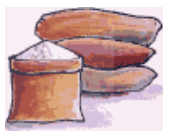Solution:
Rice consumed for 5 days in kg = 15.750, 14.850, 16.500 14.700, 17.700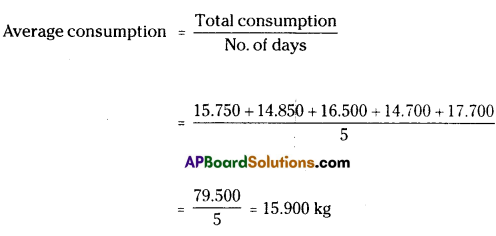∴ The average rice consumption for the 5 days = 15.900 kg.

Question 3.
In a village three different crops are cultivated in four successive years. The profit (in rupees) on the crops, per acre is shown in the table below-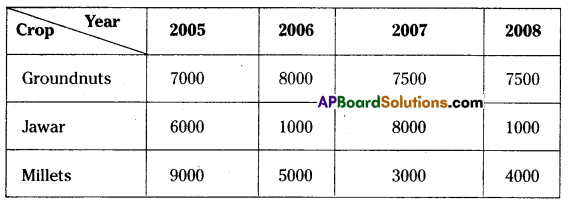(i) Calculate the mean profit for each crop over the 4 years.
(ii) Based on your answers, which crop should be cultivated in the next year?
Solution: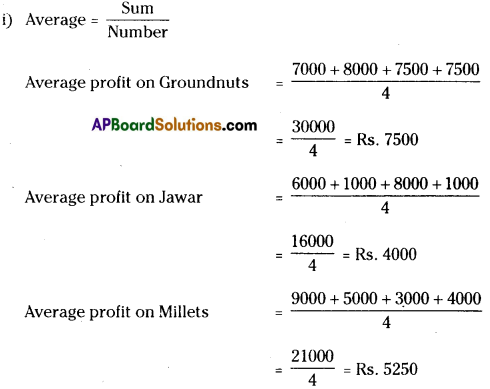(ii) As the mean profit on Groundnuts is more than the other two crops, Groundnuts may be cultivated for the next year.

Question 4.
The number of passengers who travelled in APSRTC bus from Adilabad to Nirmal in 4 trips in a day are 39, 30, 45 and 54. What is the occupancy ratio (average number of passengers travelling per trip) of the bus for the day?Solution:
Passengers travelled in 4 days 39. 30, 45 and 54
Average / Occupancy ratio =  Sum  Number = Total passengers travelled  Number of days
= 39+30+45+544=1684=42
∴ The occupancy ratio of the bus for the day = 42

Question 5.
The following table shows the marks scored by Anju, Neelesh and Lckhya in four unit tests of English.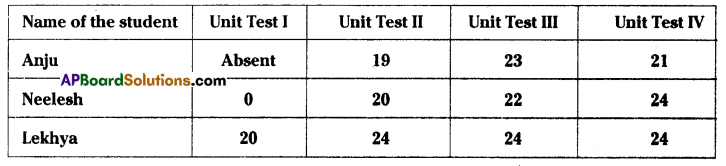(i) Find the average marks obtained by Lekhya.
(ii) Find the average marks secured by Anju. Will you divide the total marks by 3 or 4?Why?
(iii) Neelesh has given all four tests. Find the average marks secured by him. Will you divide the total marks by 3 or 4? Why?
(iv) Who performed best in the English?
Solution:
Average = Sum of the observations  Number of observations
i) Average marks obtained by Lekhya = 20+24+24+244=924 = 23
ii) Anju has given only three tests. So to find the average marks we divide by 3.
(i.e.) average = 19+23+213=633 = 21
iii) Neelesh has given 4 tests. So to find the average marks we divide by 4.
(i.e.) average = 0+20+22+244=664 = 16.5
iv) As the average marks of Lekhya is greater than the other two, we conclude that Lekhya
performed best in English.

Question 6.
Three friends went to a hotel and had breakfast to their taste, paying 16, 17 and 21 respectively
(i) Find their mean expenditure.
(ii) If they have spent 3 times the amount that they have already spent, what would their mean expenditure be?
(iii) If the hotel manager offers 50% discount, what would their mean expenditure be?
(iv) Do you notice any relationship between the change in expenditure and the change in mean expenditure.
Solution:
i) Mean expenditure =  Total expenditure  No. of persons
= 16+17+213=543 = Rs. 18

ii) Amount spent = 3 times I.e., 3 × 16; 3 × 17; 3 × 21
= Rs. 48; Rs. 51; Rs. 63
Now the average = 48+51+633=1623 = Rs. 54 = 3 x original average

iii) After 50% discount the amount spent is Half of the actual amount = 162,172,212
= Rs.8; Rs. 8.50; Rs. 10.50
Now the average = 8+8.50+10.503=27.003 = Rs .9 =  Original average 2

iv) The change In the observations is also carried out in the mean.

Question 7.
Find the mean of the first ten natural numbers.
Solution:
First ten natural numbers are 1, 2, 3, 4, 5, 6, 7, 8, 9 and 10
∴ Average =  Sum of the numbers  Number
= 1+2+3+4+5+6+7+8+9+1010=5510 = 5.5
∴ The mean of the first ten natural numbers = 5.5.

Question 8.
Find the mean of the first five prime numbers.
Solution:
First five prime numbers are 2, 3, 5, 7 and 11
Average of first five prime numbers =  Sum of the numbers  Number of primes
= 2+3+5+7+115=285 = 5.6

Question 9.
In a set of four integers, the average of the two smallest integers is 102, the average of the three smallest integers is 103, the average of all four is 104. Which is the greatest of these integers?
Solution:
Given that
The average of four lntegers = 104 =  Sum of the four integers  Number of integers
∴ The sum of the four integers = average × number
= 104 × 4
= 413
Also the average of the three smallest integers = 103 =  Sum of the three smallest integers  Number of integers
∴ The sum of the smallest three integers = average × number
= 103 × 3
= 309
∴ The greatest integer / fourth number (Sum of four Integers) – (Sum of three integers)
=416 – 309 = 107

Question 10.
Write at least two questions to find the mean, giving suitable data.
Solution:
Q – 1: The daily income of a shop-keeper during 6 days are Rs. 350, Rs. 325, Rs, 400, Rs. 450, Rs. 600, Rs. 120. Find his average daily income.
Q – 2: The number of eggs sold by a poultry during 5 days are 480, 512, 680, 720 and 1026. Find the average daily sales.

## Andhra Pradesh Board Class 7th Maths Chapter 7 Data Handling Ex 1 Textbooks for Exam Preparations

Andhra Pradesh Board Class 7th Maths Chapter 7 Data Handling Ex 1 Textbook Solutions can be of great help in your Andhra Pradesh Board Class 7th Maths Chapter 7 Data Handling Ex 1 exam preparation. The AP Board STD 7th Maths Chapter 7 Data Handling Ex 1 Textbooks study material, used with the English medium textbooks, can help you complete the entire Class 7th Maths Chapter 7 Data Handling Ex 1 Books State Board syllabus with maximum efficiency.

## FAQs Regarding Andhra Pradesh Board Class 7th Maths Chapter 7 Data Handling Ex 1 Textbook Solutions

#### Can we get a Andhra Pradesh State Board Book PDF for all Classes?

Yes you can get Andhra Pradesh Board Text Book PDF for all classes using the links provided in the above article.

## Important Terms

Andhra Pradesh Board Class 7th Maths Chapter 7 Data Handling Ex 1, AP Board Class 7th Maths Chapter 7 Data Handling Ex 1 Textbooks, Andhra Pradesh State Board Class 7th Maths Chapter 7 Data Handling Ex 1, Andhra Pradesh State Board Class 7th Maths Chapter 7 Data Handling Ex 1 Textbook solutions, AP Board Class 7th Maths Chapter 7 Data Handling Ex 1 Textbooks Solutions, Andhra Pradesh Board STD 7th Maths Chapter 7 Data Handling Ex 1, AP Board STD 7th Maths Chapter 7 Data Handling Ex 1 Textbooks, Andhra Pradesh State Board STD 7th Maths Chapter 7 Data Handling Ex 1, Andhra Pradesh State Board STD 7th Maths Chapter 7 Data Handling Ex 1 Textbook solutions, AP Board STD 7th Maths Chapter 7 Data Handling Ex 1 Textbooks Solutions,
Share: